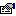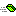## CorMath

Provides extended math functionality.

### Public:

#### Properties:

NameDescriptione (get) Represents the natural logarithmic base, specified by the constant, e.PI (get) Represents the ratio of the circumference of a circle to its diameter.

#### Methods:

NameDescriptionACos Returns the angle from a Cosine.ASin Returns the angle from a Sine.BigMul Produces the full product of two 32-bit numbers.CDeg Converts radians to degrees.Ceiling Returns the next highest whole integer value.Clamp Returns value clamped to the inclusive range of Min and Max.Cosh Returns the hyperbolic cosine of the specified angle.CRad Converts degrees to radians.DivRem Divides two integers, placing the remainder in a supplied variable.Floor Returns the next lowest whole integer value.IEEERemainder Returns the remainder resulting from the division of a specified number by another specified number.Log10 Returns a log value in a base 10 log.LogBase Returns a value in the specified base.LShift Shifts the bits of an integer left.Max Returns the maximum of two values.MaxIn Returns the maximum value from a list of values.Min Returns the minimum of two values.MinIn Returns the minimum value in a list of values.RShift Shifts the bits of an integer right.Sinh Returns the hyberbolic sine of the specified angle.Tanh Returns the hyberbolic tangent of the specified angle.

#### Remarks

The functions in this class are global. To access them simply call the functions directly as any normal function. The CorMath class does not need to be referenced.

``` Debug.Print PI
Debug.Print Max(1, 2)
```